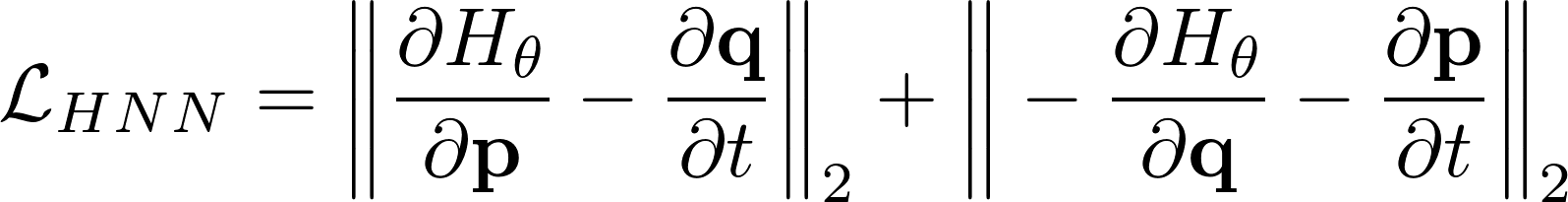# Hamiltonian Neural Networks

## Introduction

• The paper proposes a very cool idea at the intersection of deep learning and physics.

• The idea is to train a neural network architecture that builds on the concept of Hamiltonian Mechanics (from Physics) to learn physical conservation laws in an unsupervised manner.

## Hamiltonian Mechanics

• It is a branch of physics that can describe systems which follow some conservation laws and invariants.

• Consider a set of N pair of coordinates [(q1, p1), …, (qN, pN)] where q = [q1, …, qN] dnotes the position of the set of objects while p = [p1, …, pN] denotes the momentum of the set of variables.

• Together these N pairs completely describe the system.

• A scalar function H(q, p), called as the Hamiltonian is defined such that the partial derivative of H with respect to p is equal to derivative of q with respect to time t and the negative of partial derivative of H with respect to q is equal to derivative of p with respect to time t.

• This can be expressed in the form of the equation as follows:• The Hamiltonian can be tied to the total energy of the system and can be used in any system where the total energy is conserved.

## Hamiltonian Neural Network (HNN)

• The Hamiltonian H can be parameterized using a neural network and can learn conserved quantities from the data in an unsupervised manner.

• The loss function looks as follows:• The partial derivatives can be obtained by computing the in-graph gradient of the output variables with respect to the input variables.

## Observations

• For setups where the energy must be conserved exactly, (eg ideal mass-spring and ideal pendulum), the HNN learn to preserve an energy-like scalar.

• For setups where the energy need not be conserved exactly, the HNNs still learn to preserve the energy thus highlighting a limitation of HNNs.

• In case of two body problems, the HNN model is shown to be much more robust when making predictions over longer time horizons as compared to the baselines.

• In the final experiment, the model is trained on pixel observations and not state observations. In this case, two auxiliary losses are added: auto-encoder reconstruction loss and a loss on the latent space representations. Similar to the previous experiments, the HNN model makes robust predictions over much longer time horizons.